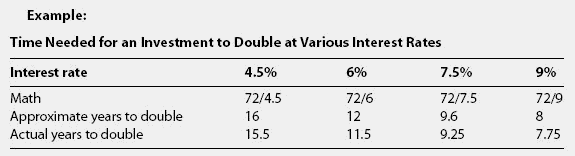# rule of 72s

## Rule of 72

A rule of thumb estimating how long it will take for an investment to double. One calculates this by dividing 72 by the rate of return. The rule of 72 is not exact, but it provides a quick look at the effects of compounding on an investment.

## rule of 72sA quick calculation of the approximate time it would take money to double when earning compound interest.Divide 72 by the interest rate to obtain the approximate answer.

Site: Follow: Share:
Open / Close